# Difference in independent effects for multivariate analysis (SPSS)

For some reason my various posts on testing differences in coefficients are fairly high in google search results. Arden Roeder writes in with another related question on this:

Good evening Dr. Wheeler,

I am a PhD candidate at the University of Oklahoma working on the final phases of data analysis for my dissertation. I found an article on your website that almost answers a question I have about a potential statistical test, and I’m hoping you might be able to help me fill in the gaps.

If you are willing to help me out, I would greatly appreciate it, and if not, I completely understand!

Here’s the setup: I have two independent variables (one measured on a 6-point Likert scale, the other on a 7-point) and six dependent variables. I have hypothesized that IV1 would be a stronger predictor of three of the DVs, and that IV2 would be a stronger predictor of the other three DVs. I ran multiple linear regression tests for each of the DVs, so I have the outputs for those. I know I can compare the standardized betas just to see strength, but what I’d like to know is how I can determine whether the difference between the beta weights is significant, and then to assess this for each of the six DVs.

From reading through your post, it seems like the fourth scenario you set up is quite close to what I’m trying to do, but I’m not sure how to translate the covariance output I have (I’m using SPSS) to what you’ve displayed here. Can I simply square the standard errors I have to get the numbers on the diagonal, and then grab the covariance from the SPSS output and solve accordingly? (I also reviewed your writing here about using the GLM procedure as well, but can’t seem to align my outputs with your examples there either.)

Here’s a sample of the numbers I’m working with: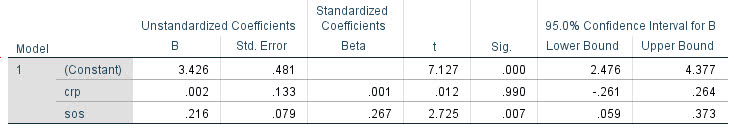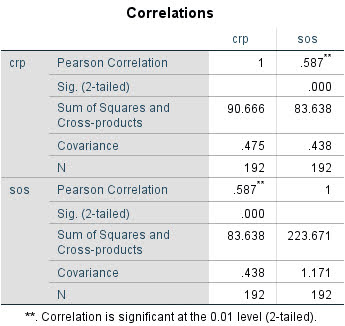Any insights you can offer on 1) whether this is the right test to give me the answers I’m looking for about whether the betas are significantly different and 2) how to set up and interpret the results correctly would be a tremendous help.

For 1, yes I think this is an appropriate way to set up the problem. For 2, if sticking to SPSS it is fairly simple syntax in GLM:

``````*****************************.
*Contrasts with X1 - X2 effect across the variables.
GLM Y1 Y2 Y3 Y4 Y5 Y6 WITH X1 X2
/DESIGN=X1 X2
/PRINT PARAMETER
/LMATRIX = "T1"
X1  1
X2 -1.
*****************************.``````

To get this to do the standardized coefficients, Z-score your variables before the GLM command (this is assuming you are estimating a linear model, and not a non-linear model like logit/Poisson). (I have full simulation SPSS code at the end of the post illustrating.)

Note that when I say the covariance between beta coefficients, this is NOT the same thing as the covariance between the original metrics. So the correlation matrix for X1 vs X2 here does not give us the information we need.

For 2, the reporting part, you can see the Contrast results K matrix table in SPSS. I would just transpose that table, make a footnote/title this is testing X1 – X2, and then just keep the columns you want. So here is the original SPSS contrast table for this result: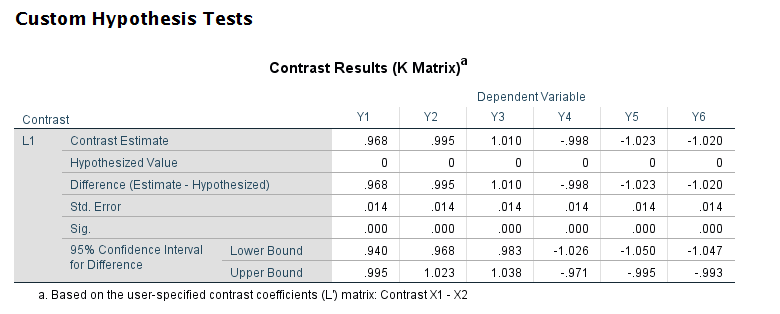And here is how I would clean up the table and report the tests: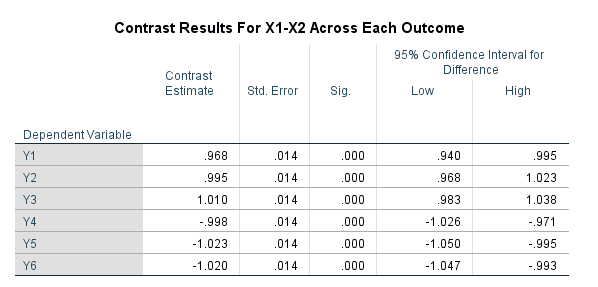# SPSS Simulation Code

Here I just simulate independent X’s, and give the Y’s consistent effects. You could also simulate multivariate data with specified correlations if you wanted to though.

``````****************************************************.
* Simulated data to illustrate coefficient diff.
* tests.
SET SEED 10.
INPUT PROGRAM.
LOOP #i = 1 TO 10000.
COMPUTE Id = #i.
END CASE.
END LOOP.
END FILE.
END INPUT PROGRAM.

COMPUTE X1 = RV.NORMAL(0,1).
COMPUTE X2 = RV.NORMAL(0,1).
COMPUTE Y1 = 1.5*X1 + 0.5*X2 + RV.NORMAL(0,1).
COMPUTE Y2 = 1.5*X1 + 0.5*X2 + RV.NORMAL(0,1).
COMPUTE Y3 = 1.5*X1 + 0.5*X2 + RV.NORMAL(0,1).
COMPUTE Y4 = 0.5*X1 + 1.5*X2 + RV.NORMAL(0,1).
COMPUTE Y5 = 0.5*X1 + 1.5*X2 + RV.NORMAL(0,1).
COMPUTE Y6 = 0.5*X1 + 1.5*X2 + RV.NORMAL(0,1).
EXECUTE.

*Contrasts with X1 - X2 effect across the variables.
GLM Y1 Y2 Y3 Y4 Y5 Y6 WITH X1 X2
/DESIGN=X1 X2
/PRINT PARAMETER
/LMATRIX = "Contrast X1 - X2"
X1  1
X2 -1.

*And here is an example stacking equations and using EMMEANS.
*Stacking equation approach, can work for various.
*Generalized linear models, etc.
VARSTOCASES
/MAKE Y FROM Y1 TO Y6
/Index Outcome.

GENLIN Y BY Outcome WITH X1 X2
/MODEL Outcome X1 X2 Outcome*X1 Outcome*X2
/CRITERIA COVB=ROBUST
/REPEATED SUBJECT=Id CORRTYPE=UNSTRUCTURED
/EMMEANS TABLES=Outcome CONTROL= X1(1) X2(-1).
****************************************************.``````

# Marginal effects vs Wald tests (Stata)

Calli Cain, a criminologist from FAU asks:

What is the best method to examine whether there are group differences (e.g., gender, race) in the effects of several variables on binary outcomes (using logistic regression)? For example – if you want to look at the gendered effects of different types of trauma experiences on subsequent adverse behaviors (e.g., whether participant uses drugs, alcohol, has mental health diagnosis, has attempted suicide). Allison (1999) cautions against using Equality of Coefficients tests to look at group differences between regression coefficients like we might with OLS regression. If you wanted to look at the differences between a lot of predictors (n= 16) on various outcomes (n=6) – what would be best way to go about it (I know using interaction terms would be good if you were just interested in say gender differences of one or two variables on the outcome). Someone recommended comparing marginal effects through average discrete changes (ADCs) or discrete changes at representative values (DCRs) – which is new to me. Would you agree with this suggestion?

When I am thinking about should I use method X or method Y type problems, I think about the specific value I want to estimate first, and then work backwards about the best method to use to get that estimate. So if we are talking about binary endpoints such as uses drugs (will go with binary for now, but my examples will readily extend to say counts or real valued outcomes), there are only generally two values people are interested in; say the change in probability is 5% given some input (absolute risk change, e.g. 10% to 5%), or a relative risk change such as X decreases the overall relative risk by 20% (e.g. 5% to 4%).

The former, absolute change in probabilities, is best accomplished via various marginal effects or average discrete changes as Calli says. Most CJ examples I am aware of I think these make the most sense to focus on, as they translate more directly to costs and benefits. Focusing on the ratio’s sometimes makes sense, such as in case-control studies, or if you want to extrapolate coefficient estimates to a very different sample. Or if you are hyper focused on theory and the underlying statistical model.

Will show an example in Stata using simulated data to illustrate the differences. Stata is very nice to work with different types of marginal estimates. Here I generate data with three covariates, female/males, and then some interactions. Note the covariate x1 has the same effect for males/females, x2 and x3 though have countervailing effects (females it decreases, males it increases the probability).

``````* Stata simulation to show differences in Wald vs Margins
clear
set more off
set seed 10
set obs 5000
generate female = rbinomial(1,0.5)
generate x1 = rnormal(0,1)
generate x2 = rnormal(0,1)
generate x3 = rnormal(0,1)

* x1 same effect, x2/x3 different across groups
generate logit = -0.1 + -2.8*female + 1.1*(x1 + x2 + x3) + -1.5*female*(x2 + x3)
generate prob = 1/(1 + exp(-1*logit))
generate y = rbinomial(1,prob)
drop logit prob``````

I intentionally generated the groups so females/males have quite different baseline probabilities for the outcome y here – something that happens in real victim data in criminology.

``````* Check out marginal differences
tabstat y, by(female)``````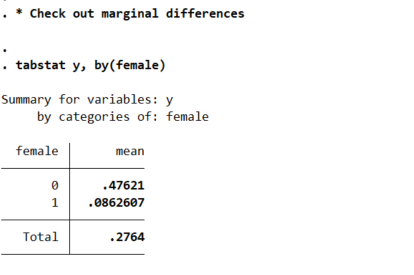So you can see males have the proportion of the outcome near 50% in the sample, whereas females are under 10%. So Calli is basically interested in the case below, where we estimate all pairwise interactions, so have many coefficient differences to test on the right hand side.

``````* Estimate model with interactions (linear coefficients)
logistic y i.female x1 x2 x3 i.female#(c.x1 c.x2 c.x3), coef``````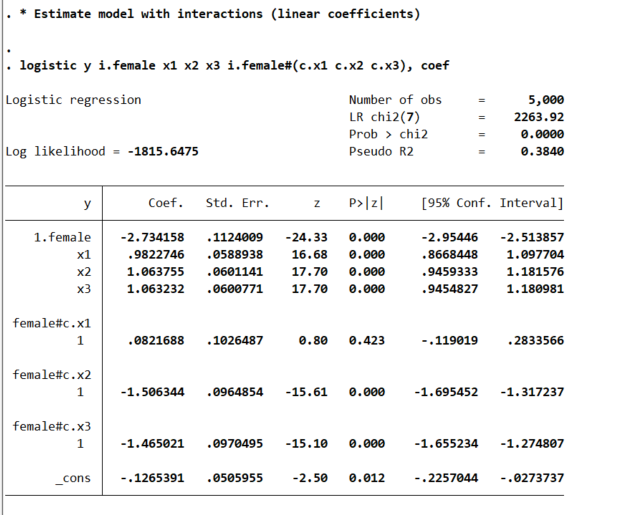This particular model does the Wald tests for the coefficient differences just by the way we have set up the model. So the interaction effects test for differences from the baseline model, so can see the interaction for x1 is not stat significant, but x2/x3 are (as they should be). But if you are interested in the marginal effects, one place to start is with derivatives directly, and Stata automatically for logit models spits out probabilities:

``````* Marginal effects
margins female, dydx(x1 x2 x3)
* x1 is the same linear effect, but margins are quite different``````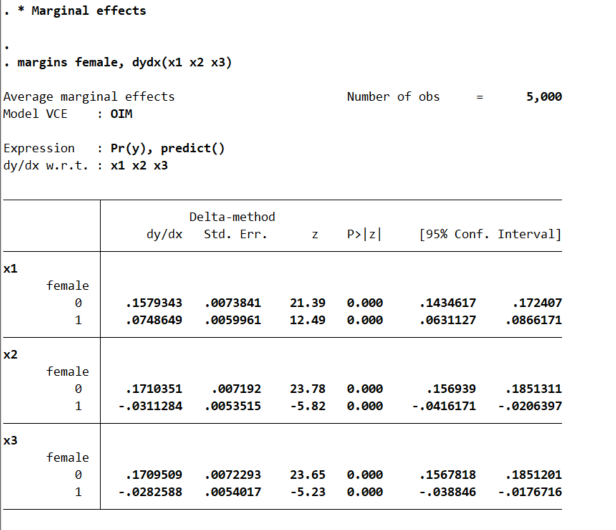So even though I made the underlying effect for x1 equal between males/females for the true underlying data generation process, you can see here the marginal derivative is much smaller for females. This is the main difference between Wald tests and margins.

This is ok though for many situations. Say x1 is a real valued treatment, such as Y is victimization in a sample of high risk youth, and x1 is hours given for a summer job. We want to know the returns of expanding the program – here the returns are higher for males than females due to the different baseline probabilities of risk between the two. This is true even if the relative effect of summer job hours is the same between the two groups.

Again Stata is very convenient, and we can test for the probability differences in males/females by appending `r.` to the front of the margins command.

``````* can test difference contrast in groups
margins r.female, dydx(x1 x2 x3)``````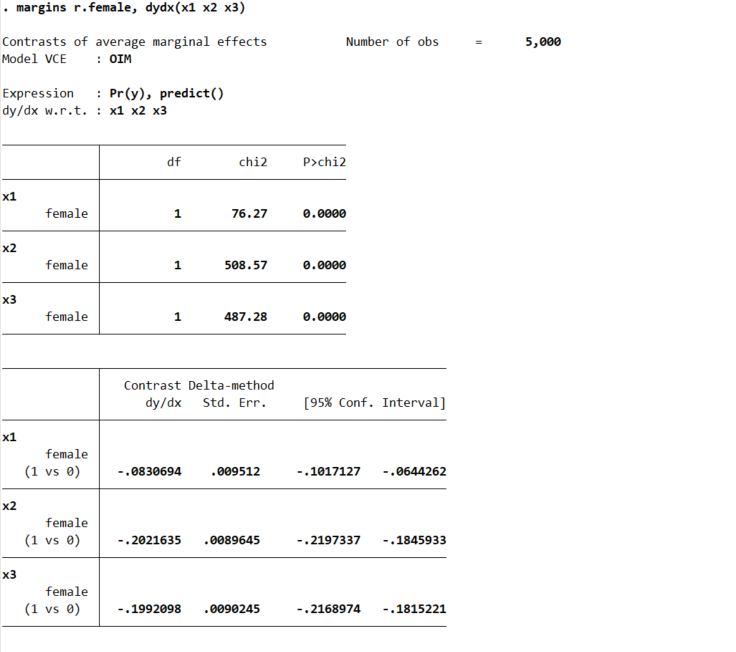But the marginal derivative can be difficult to interpret – it is the average slope, but what does that mean exactly? So I like evaluating at fixed points of the continuous variable. Going back to our summer job hours example, you could evaluate going from 0 to 50 hours, or going from 50 to 100, or 0 to 100, etc. and see the average returns in terms of reductions in the probability of trauma. Here because I simulated the data to be a standard normal, I just go from -1 to 0 to 1:

``````* Probably easier to understand at particular x1 values
margins female, at(x1=(-1(1)1))``````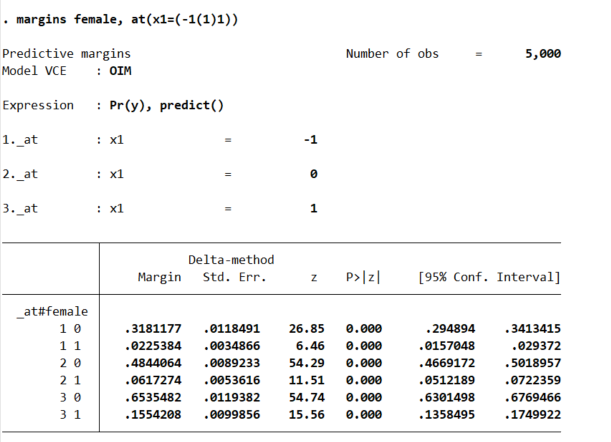So that table is dense, but it says when x1=-1, females have a probability of y of 2%, and males have a probability of y of 32%. Now go up the ladder to x1=0, females have a probability of 6% and males have a probability of 48%. So a discrete change of 4% for females and 16% for males. If we want to generate an interval around that discrete change effect:

``````* Can test increases one by one
margins female, at(x1=(-1 0 1))   contrast(atcontrast(ar) effects marginswithin)``````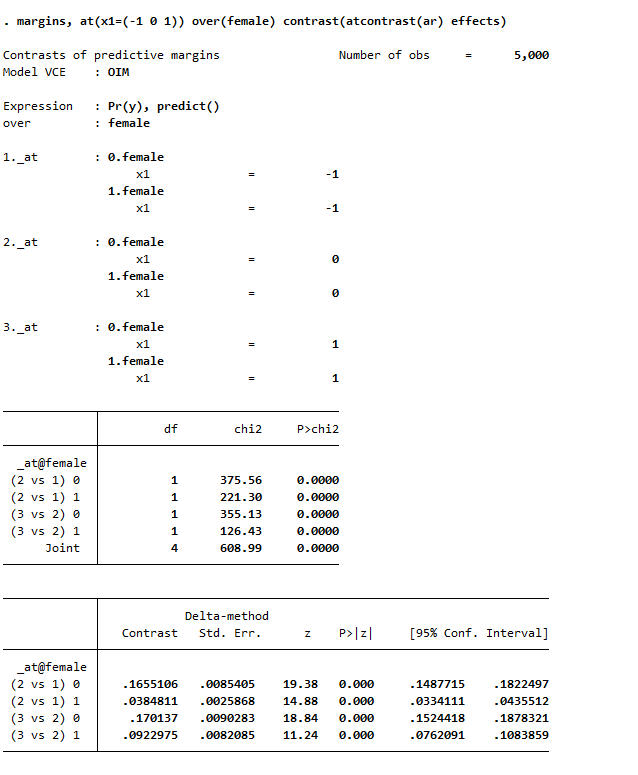See, isn’t Stata’s margins command so nice! (For above, it actually may make more sense to use `margins , at(x1=(-1 0 1)) over(female) contrast(atcontrast(ar) effects)`. Margins estimates the changes over the whole sample and averages filling in certain values, with over it only does the averaging within each group on over.) And finally we can test the difference in difference, to see if the discrete changes in males females from going from -1 to 0 to 1 are themselves significant:

``````* And can test increases of males vs females
margins r.female, at(x1=(-1(1)1)) contrast(atcontrast(ar))``````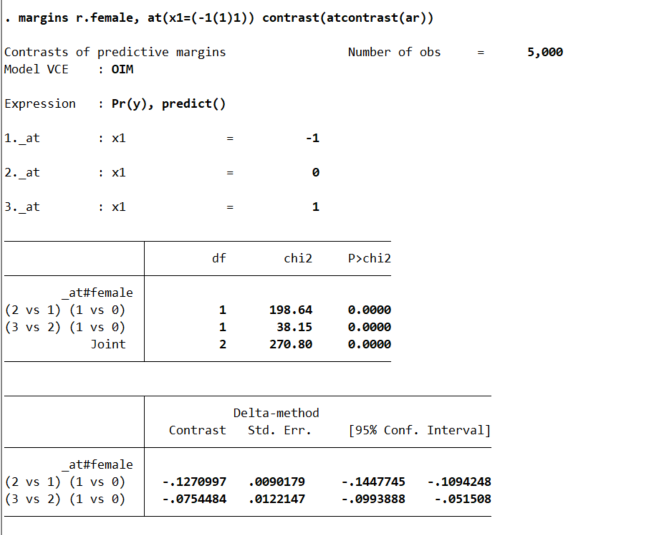So the increase in females is 13% points smaller than the increase in males going from -1 to 0, etc.

So I have spent alot of time on the probabilities so far. I find them much easier to interpret, and I do not care so much about the fact that it doesn’t necessarily say the underlying DGP is different from males/females. But many people are interested in the odds ratios (say in case-control studies). Or generalizing to a different sample, say this is a low risk sample of females, and you want to generalize the odds ratio’s to a higher risk sample with a baseline more around 50%. Then looking at the odds ratio may make more sense.

Or so far I have not even gotten to Calli’s main point, how to test many of these effects for no differences at once. There I would just suggest the likelihood ratio test (which does not have the problems with the Wald tests on the coefficients and that the variance estimates may be off):

``````* Estimate restricted model
logistic y ibn.female c.x1 c.x2 c.x3, coef noconstant
estimates store restrict

* Another way to do the full interaction model
* More like separate male and female
logistic y ibn.female ibn.female#(c.x1 c.x2 c.x3), coef noconstant
estimates store full

* LRT test between models
lrtest restrict full``````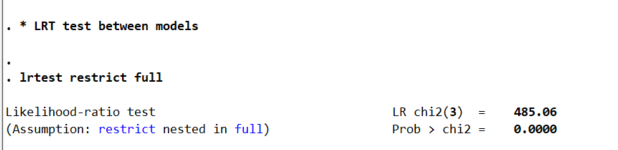So here as expected, one rejects the null that the restricted model is a better fit to the data. But this is pretty uninformative – I rather just go to the more general model and quantify the differences.

So if you really want to look at the odds ratios, we can do that using `lincom` post our full interaction model:

``logistic y i.female ibn.female#(c.x1 c.x2 c.x3), coef noconstant``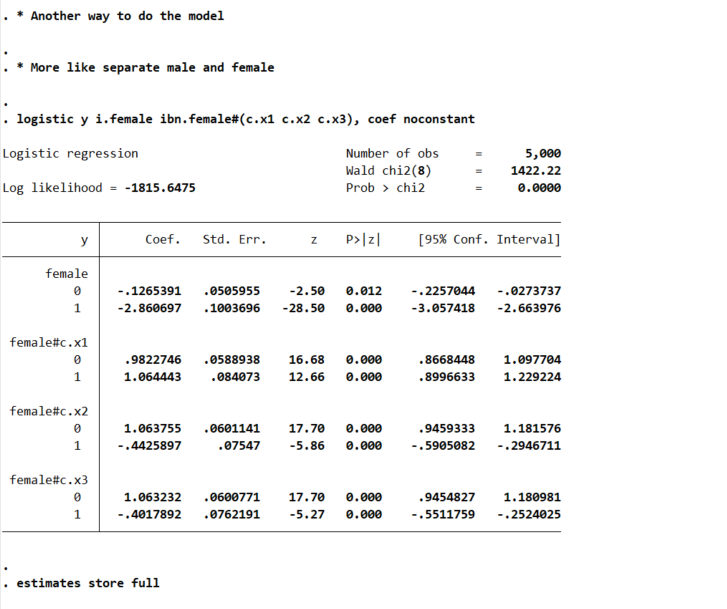And here is an example post Wald test for equality:

``lincom 0.female#c.x1 - 1.female#c.x1``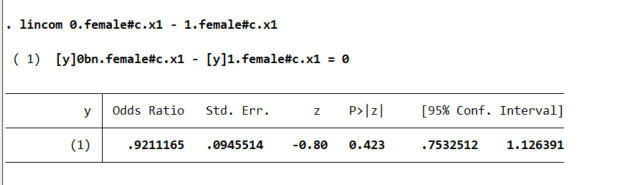You may ask where does this odd’s ratio of 0.921 come from? Well, way back in our first full model, the interaction term for `female*x1` is `0.0821688`, and `exp(-0.0821688)` equals that odds ratio and has the same p-value as the original model I showed. And so you can see that the x1 effect is the same across each group. But estimating the other contrasts is not:

``lincom 0.female#c.x2 - 1.female#c.x2``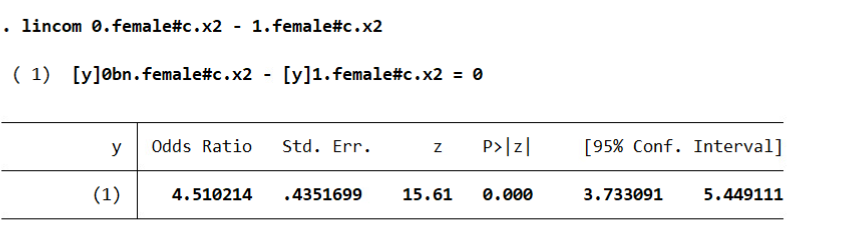And Stata defaults this to estimating a difference in the odds ratio (as far as I can tell you can’t tell Stata to do the linear coefficient after logit, would need to change the model to `glm y x, family(binomial) link(logit)` to do the linear tests).

I honestly don’t know how to really interpret this – but I have been asked for it several different times by clients. Maybe they know better than me, but I think it is more to do with people just expect to be dealing with odds ratios after a logistic regression.

So we can coerce margins to give us odds ratios:

``````* For the odds ratios
quietly margins female, at(x3=(-1(0.1)1)) expression(exp(predict(xb)))
marginsplot , yscale(log) ylabel(0.125 0.25 0.5 1 2 4 8)``````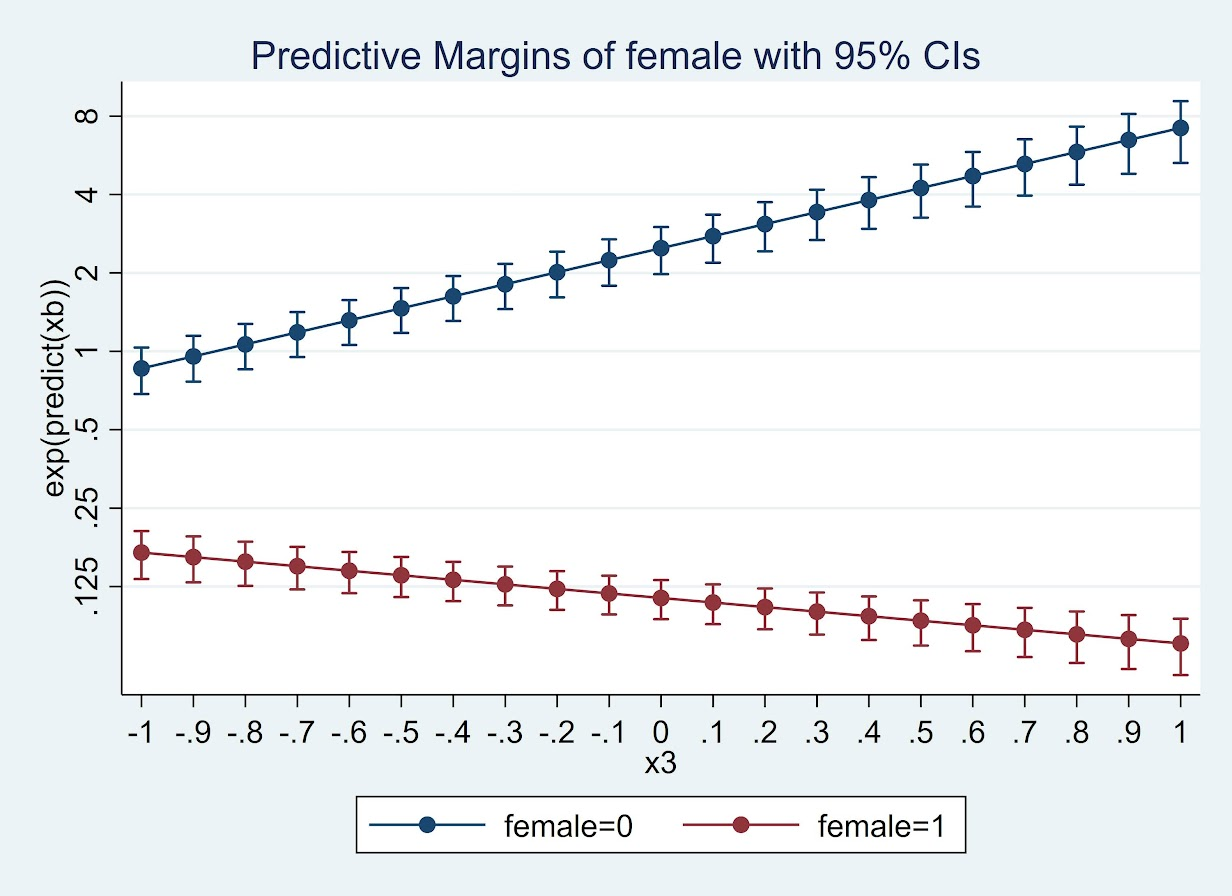Or give us the differences in the odds ratio:

``````* For the contrast in the OR
quietly margins r.female, at(x3=(-1(0.1)1)) expression(exp(predict(xb)))
marginsplot``````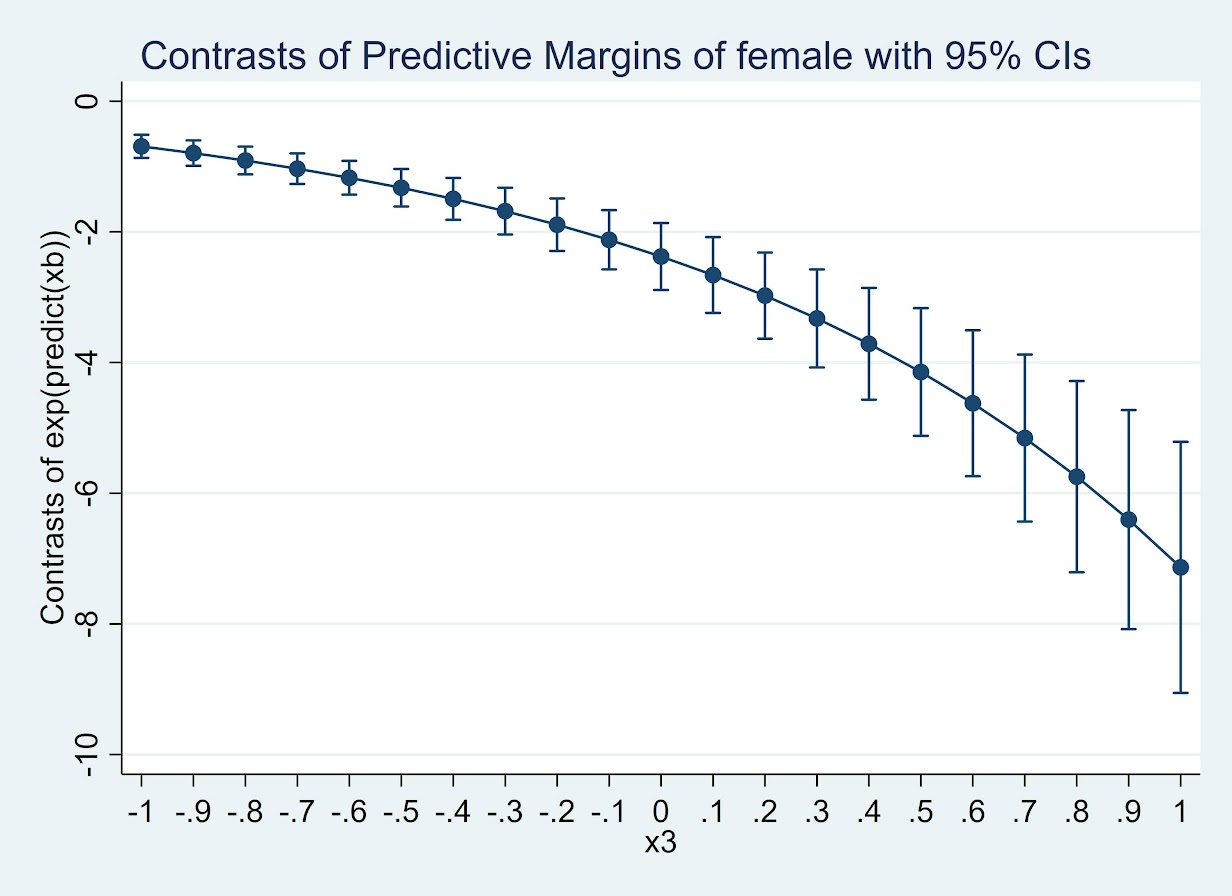(Since it is a negative number cannot be drawn on a log scale.) But again I find it much easier to wrap my head around probabilities:

``````* For the probabilities
quietly margins female, at(x3=(-2(0.1)2))
marginsplot``````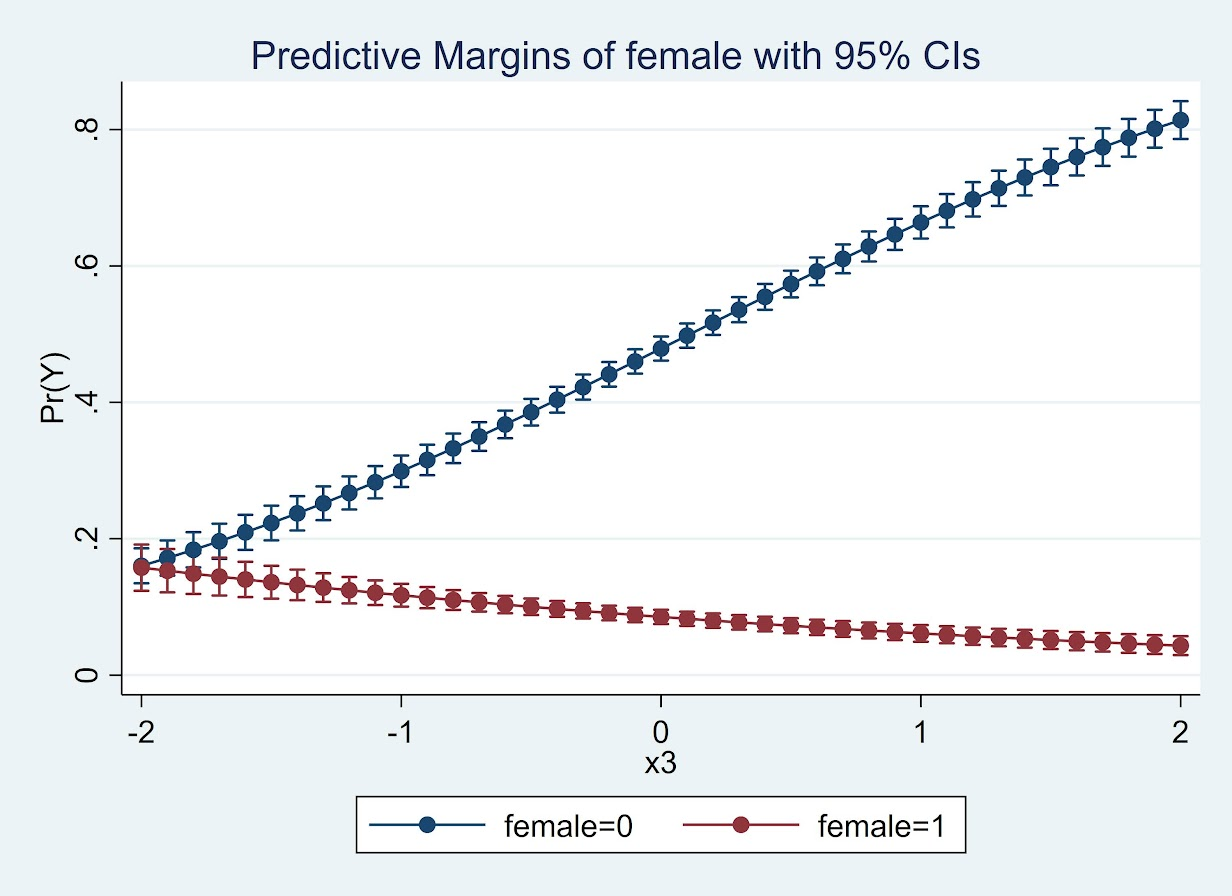So here while x3 increases, for males it increases the probability and females it decreases. The female decrease is smaller due to the smaller baseline risk in females.

So while Calli’s original question was how to do this reasonably for many different contrasts, I would prefer the actual empirical estimates of the differences. Doing a single contrast among a small number of representative values over many variables and placing in a table/graph I think is the best way to reduce the complexity.

I just don’t find the likelihood ratio tests for all equalities that informative, and for large samples they will almost always say the more flexible model is better than the restricted model.

We estimate models to actually look at the quantitative values of those estimates, not to do rote hypothesis testing.

# Wald tests via statsmodels (python)

The other day on crossvalidated a question came up about interpreting treatment effect differences across different crime types. This comes up all the time in criminology research, especially interventions intended to reduce crime.

Often times interventions are general and may be expected to reduce multiple crime types, e.g. hot spots policing may reduce both violent crimes and property crimes. But we do not know for sure – so it makes sense to fit models to check if that is the case.

For crimes that are more/less prevalent, this is a case in which fitting Poisson/Negative Binomial models makes alot of sense, since the treatment effect is in terms of rate modifiers. The crossvalidated post shows an example in R. In the past I have shown how to stack models and do these tests in Stata, or use seemingly unrelated regression in Stata for generalized linear models. Here I will show an example in python using data from my dissertation on stacking models and doing Wald tests.

The above link to github has the CSV file and metadata to follow along. Here I just do some upfront data prep. The data are crime counts at intersections/street segments in DC, across several different crime types and various aspects of the built environment.

``````# python code to stack models and estimate wald tests
import pandas as pd
import numpy as np
import statsmodels.formula.api as smf
import patsy
import itertools

# Use dissertation data for multiple crimes
#https://github.com/apwheele/ResearchDesign/tree/master/Week02_PresentingResearch

# only keep a few independent variables to make it simpler
crime = ['OffN1','OffN3','OffN5','OffN7','OffN8','OffN9'] #dropping very low crime counts
x = ['CFS1','CFS2'] #311 calls for service
data = data[crime + x].copy()
data.reset_index(inplace=True)

# Stack the data into long format, so each crime is a new row
data_long = pd.wide_to_long(data, 'OffN',i='MarID',j='OffCat').reset_index()``````

And here you can see what the data looks like before (wide) and after (long). I am only fitting one covariate here (detritus 311 calls for service, see my paper), which is a measure of disorder in an area.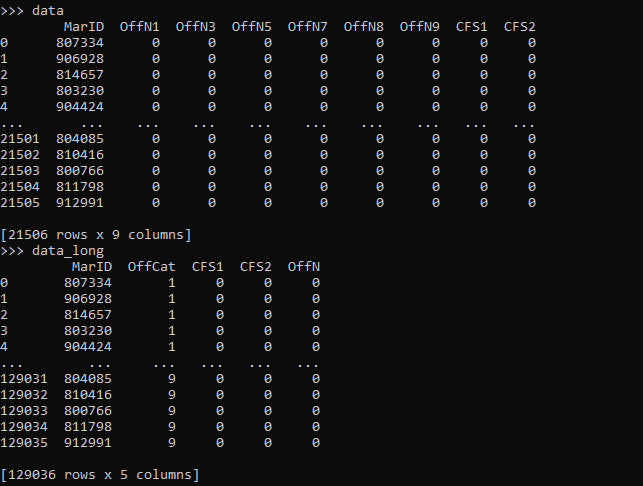For reference the offense categories are below, and I drop homicide/arson/sex abuse due to very low counts.

``````'''
Offense types in the data
OffN2   Arson #drop
OffN3   Burglary
OffN4   Homicide #drop
OffN5   Robbery
OffN6   Sex Abuse #drop
OffN7   Stolen Auto
OffN8   Theft
OffN9   Theft from Auto
'''``````

Now we can fit our stacked negative binomial model. I am going right to negative binomial, since I know this data is overdispersed and Poisson is not a good fit. I account for the clustering induced by stacking the equations, although with such a large sample it should not be a big deal.

``````# Fit a model with clustered standard errors
covp = {'groups': data_long['MarID'],'df_correction':True}
nb_mod = smf.negativebinomial('OffN ~ C(OffCat) + CFS1:C(OffCat) - 1',data_long).fit(cov_type='cluster',cov_kwds=covp)
print(nb_mod.summary())``````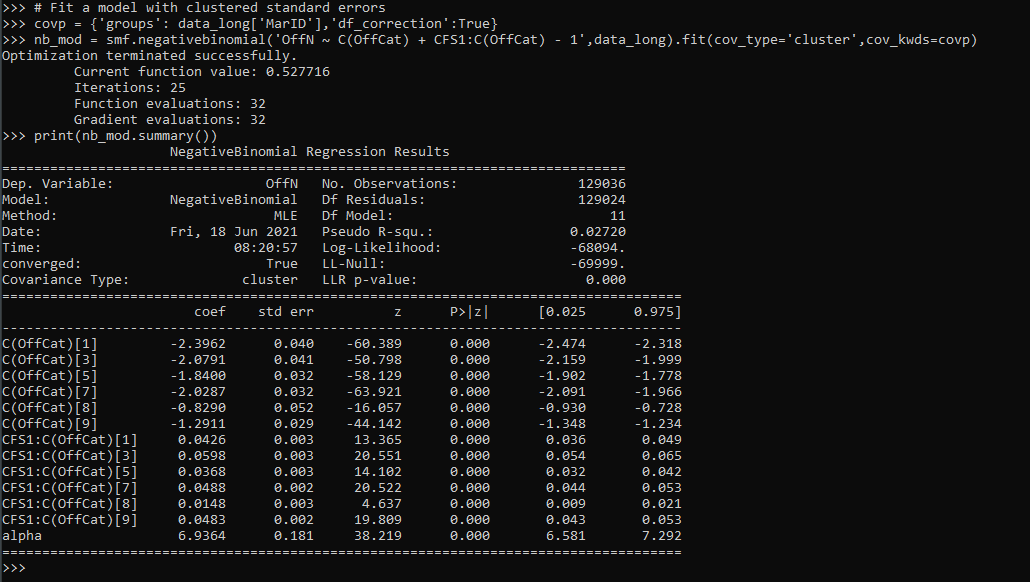So this is close to the same if you fit a separate regression for each crime type. You get an intercept for each crime type (the `C(OffCat[?])` coefficients), as well as a varying treatment effect for calls for service across each crime type, e.g. `CFS1:C(OffCat)` is the effect of 311 calls to increase Assaults, `CFS1:C(OffCat)` is to increase Burglaries, etc.

One limitation of this approach is that alpha here is constrained to be equal across each crime type. (Stata can get around this, either with the stacked equation fitting an equation for alpha based on the offense categories, or using the suest command.) But it is partly sweating the small stuff, the mean equation is the same. (So it may also make sense to not worry about clustering and fit a robust covariance estimate to the Poisson equation.)

Now onto the hypothesis tests. Besides seeing whether any individual coefficient equals 0, we may also have two additional tests. One is whether the treatment effect is equal across the different crime types. Here is how you do that in python for this example:

``````# Conduct a Wald test for equality of multiple coefficients
x_vars = nb_mod.summary2().tables.index
wald_str = ' = '.join(list(x_vars[6:-1]))
print(wald_str)
wald_test = nb_mod.wald_test(wald_str) # joint test
print(wald_test)``````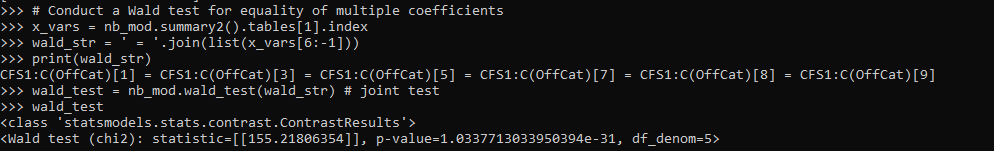Given the large sample size, even though all of the coefficients for garbage 311 calls are very similar (mostly around 0.3~0.5), this joint test says they do not all equal each other.

So the second hypothesis people are typically interested in is whether the coefficients all equal zero, a joint test. Here is how you do that in python statsmodels. They have a convenient function `.wald_test_terms()` to do just this, but I also show how to construct the same string and use `.wald_test()`.

``````# Or can do test all join equal 0
nb_mod.wald_test_terms()
# To replicate what wald_test_terms is doing yourself
all_zero = [x + '= 0' for x in x_vars[6:-1]]
nb_mod.wald_test(','.join(all_zero))``````

So we have established that when testing the equality between the coefficients, we reject the null. But this does not tell us which contrasts are themselves different and the magnitude of those coefficient differences. We can use .`t_test()` for that. (Which is the same as a Wald test, just looking at particular contrasts one by one.)

``````# Pairwise contrasts of coefficients
# To get the actual difference in coefficients
wald_li = []
for a,b in itertools.combinations(x_vars[6:-1],2):
wald_li.append(a + ' - ' + b + ' = 0')

wald_dif = ' , '.join(wald_li)

dif = nb_mod.t_test(wald_dif)
print(dif)

# c's correspond to the wald_li list
res_contrast = dif.summary_frame()
res_contrast['Test'] = wald_li
res_contrast.set_index('Test', inplace=True)
print(res_contrast)``````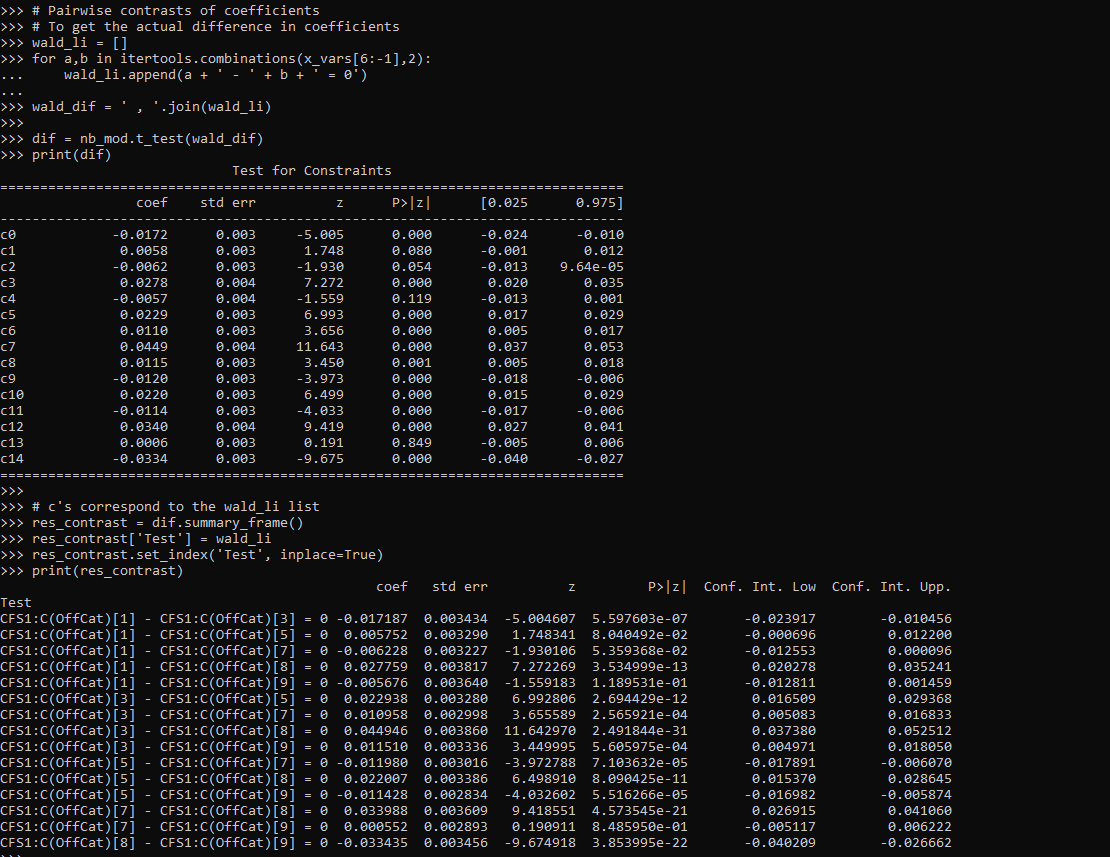You can see the original t-test table does not return a nice set of strings illustrating the actual test. So I show here how they correspond to a particular hypothesis. I actually wrote a function to give nice labels given an input test string (what you would submit to either `.t_test()` or `.wald_test()`).

``````# Nicer function to print out the actual tests it interprets as
# ends up being 1 - 3, 3 - 5, etc.
def nice_lab_tests(test_str,mod):
# Getting exogenous variables
x_vars = mod.summary2().tables.index
# Patsy getting design matrix and constraint from string
di = patsy.DesignInfo(x_vars)
const_mat = di.linear_constraint(test_str)
r_mat = const_mat.coefs
c_mat = list(const_mat.constants)
# Loop over the tests, get non-zero indices
# Build the interpreted tests
lab = []
for i,e in enumerate(c_mat):
lm = r_mat[i,:] #single row of R matrix
nz = np.nonzero(lm).tolist() #only need non-zero
c_vals = lm[nz].tolist()
v_labs = x_vars[nz].tolist()
fin_str = ''
in_val = 0
for c,v in zip(c_vals,v_labs):
# 1 and -1 drop values and only use +/-
if c == 1:
if in_val == 0:
fin_str += v
else:
fin_str += ' + ' + v
elif c == -1:
if in_val == 0:
fin_str += '-' + v
else:
fin_str += ' - ' + v
else:
if in_val == 0:
fin_str += str(c) + '*' + v
else:
if c > 0:
sg = ' + '
else:
sg = ' - '
fin_str += sg + str(np.abs(c)) + '*' + v
in_val += 1
fin_str += ' = ' + str(e) #set equality at end
lab.append(fin_str)
return lab``````

So if we look at our original `wald_str`, this converts the equality tests into a series of difference tests against zero.

``````# Wald string for equality across coefficients
# from earlier
lab_tests = nice_lab_tests(wald_str,nb_mod)
print(lab_tests)``````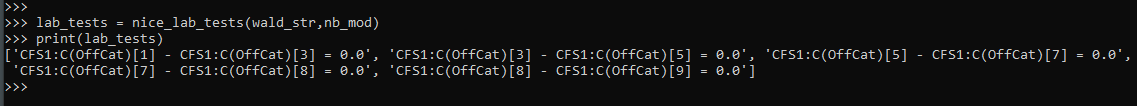And this function should work for other inputs, here is another example:

``````# Additional test to show how nice_lab_tests function works
str2 = 'CFS1:C(OffCat) = 3, CFS1:C(OffCat) = CFS1:C(OffCat)'
nice_lab_tests(str2,nb_mod)``````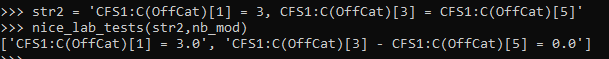Next up on the agenda is a need to figure out `.get_margeff()` a bit better for these statsmodels (or perhaps write my own closer to Stata’s implementation).

# Some more testing coefficient contrasts: Multinomial models and indirect effects

Testing the equality of two coefficients is one of my more popular posts. This is a good thing — often more interesting hypotheses are to test two parameters against each other, as opposed to a strict null hypothesis of a coefficient against zero. Every now an then I get questions about applying this idea to new situations in which it is not always straightforward how to figure out. So here are a few examples using demonstration R code.

# Multinomial Models

One question I received about applying the advice was to test coefficients across different contrasts in multinomial models. It may not seem obvious, but the general approach of extracting out the coefficients and the covariance between those estimates works the same way as most regression equations.

So in a quick example in R:

``````library(nnet)
data(mtcars)
library(car)

mtcars\$cyl <- as.factor(mtcars\$cyl)
mtcars\$am <- as.factor(mtcars\$am)
mod <- multinom(cyl ~ am + hp, data=mtcars, Hess=TRUE)
summary(mod)``````

And the estimates for `mod` are:

``````> summary(mod)
Call:
multinom(formula = cyl ~ am + hp, data = mtcars)

Coefficients:
(Intercept)       am1        hp
6   -42.03847  -3.77398 0.4147498
8   -92.30944 -26.27554 0.7836576

Std. Errors:
(Intercept)       am1        hp
6    27.77917  3.256003 0.2747842
8    31.93525 46.854100 0.2559052

Residual Deviance: 7.702737
AIC: 19.70274 ``````

So say we want to test whether the `hp` effect is the same for `6` cylinders vs `8` cylinders. To test that, we just grab the covariance and construct our test:

``````#Example constructing test by hand
v <- vcov(mod)
c <- coef(mod)
dif <- c[1,3] - c[2,3]
se <- sqrt( v[3,3] + v[6,6] - 2*v[3,6])
z <- dif/se
#test stat, standard error, and two-tailed p-value
dif;se;2*(1 - pnorm(abs(z)))``````

Which we end up with a p-value of `0.0002505233`, so we would reject the null that these two effects are equal to one another. Note to get the variance-covariance estimates for the parameters you need to set `Hess=TRUE` in the `multinom` call.

Another easier way though is to use the `car` libraries function `linearHypothesis` to conduct the same test:

``````> linearHypothesis(mod,c("6:hp = 8:hp"),test="Chisq")
Linear hypothesis test

Hypothesis:
6:hp - 8:hp = 0

Model 1: restricted model
Model 2: cyl ~ am + hp

Df  Chisq Pr(>Chisq)
1
2  1 13.408  0.0002505 ***
---
Signif. codes:  0 ‘***’ 0.001 ‘**’ 0.01 ‘*’ 0.05 ‘.’ 0.1 ‘ ’ 1``````

You can see although this is in terms of a Chi-square test, it results in the same p-value. The Wald test however can be extended to testing multiple coefficient equalities, and a popular one for multinomial models is to test if any coefficients change across different levels of the dependent categories. The idea behind that test is to see if you can collapse that category with another that is equivalent.

To do that test, I created a function that does all of the contrasts at once:

``````#Creating function to return tests for all coefficient equalities at once
all_tests <- function(model){
v <- colnames(coef(model))
d <- rownames(coef(model))
allpairs <- combn(d,2,simplify=FALSE)
totn <- length(allpairs) + length(d)
results <- data.frame(ord=1:totn)
results\$contrast <- ""
results\$test <- ""
results\$Df <- NULL
results\$Chisq <- NULL
results\$pvalue <- NULL
iter <- 0
for (i in allpairs){
iter <- iter + 1
l <- paste0(i,":",v)
r <- paste0(i,":",v)
test <- paste0(l," = ",r)
temp_res <- linearHypothesis(model,test,test="Chisq")
results\$contrast[iter] <- paste0(i," vs ",i)
results\$test[iter] <- paste(test,collapse=" and ")
results\$Df[iter] <- temp_res\$Df
results\$Chisq[iter] <- temp_res\$Chisq
results\$pvalue[iter] <- temp_res\$Pr
}
ref <- model\$lab[!(model\$lab %in% d)]
for (i in d){
iter <- iter + 1
test <- paste0(i,":",v," = 0")
temp_res <- linearHypothesis(model,test,test="Chisq")
results\$contrast[iter] <- paste0(i," vs ",ref)
results\$test[iter] <- paste(test,collapse=" and ")
results\$Df[iter] <- temp_res\$Df
results\$Chisq[iter] <- temp_res\$Chisq
results\$pvalue[iter] <- temp_res\$Pr
}
return(results)
}``````

Not only does this construct the test of the observed categories, but also tests whether each set of coefficients is simultaneously zero, which is the appropriate contrast for the referent category.

``````> all_tests(mod)
ord contrast                                                            test Df        Chisq       pvalue
1   1   6 vs 8 6:(Intercept) = 8:(Intercept) and 6:am1 = 8:am1 and 6:hp = 8:hp  3    17.533511 0.0005488491
2   2   6 vs 4                    6:(Intercept) = 0 and 6:am1 = 0 and 6:hp = 0  3     5.941417 0.1144954481
3   3   8 vs 4                    8:(Intercept) = 0 and 8:am1 = 0 and 8:hp = 0  3 44080.662112 0.0000000000``````

User beware of multiple testing with this, as I am not sure as to the appropriate post-hoc correction here when examining so many hypotheses. This example with just three is obviously not a big deal, but with more categories you get n choose 2, or `(n*(n-1))/2` total contrasts.

# Testing the equality of multiple indirect effects

Another example I was asked about recently was testing whether you could use the same procedure to calculate indirect effects (popular in moderation and mediation analysis). Those end up being a bit more tricky, as to define the variance and covariance between those indirect effects we are not just dealing with adding and subtracting values of the original parameters, but are considering multiplications.

Thus to estimate the standard error and covariance parameters of indirect effects folks often use the delta method. In R using the `lavaan` library, here is an example (just taken from a code snippet Yves Rosseel posted himself), to estimate the variance-covariance matrix model defined indirect parameters.

``````#function taken from post in
library(lavaan)
vcov.def <- function(model){
m <- model
orig <- vcov(m)
free <- m@Fit@x
jac <- lavaan:::lavJacobianD(func = m@Model@def.function, x = free)
vcov_def <- jac %*% orig %*% t(jac)
estNames <- subset(parameterEstimates(m),op==":=")
row.names(vcov_def) <- estNames\$lhs
colnames(vcov_def) <- estNames\$lhs
#I want to print the covariance table estimates to make sure the
#labels are in the correct order
estNames\$se2 <- sqrt(diag(vcov_def))
estNames\$difSE <- estNames\$se - estNames\$se2
print(estNames[,c('lhs','se','se2','difSE')])
print('If difSE is not zero, labels are not in right order')
return(vcov_def)
}``````

Now here is an example of testing individual parameter estimates for indirect effects.

``````set.seed(10)
n <- 100
X1 <- rnorm(n)
X2 <- rnorm(n)
X3 <- rnorm(n)
M <- 0.5*X1 + 0.4*X2 + 0.3*X3 + rnorm(n)
Y <- 0.1*X1 + 0.2*X2 + 0.3*X3 + 0.7*M + rnorm(n)
Data <- data.frame(X1 = X1, X2 = X2, X3 = X3, Y = Y, M = M)
model <- ' # direct effect
Y ~ X1 + X2 + X3 + d*M
# mediator
M ~ a*X1 + b*X2 + c*X3
# indirect effects
bd := b*d
cd := c*d
'
model_SP.fit <- sem(model, data = Data)
summary(model_SP.fit)

#now apply to your own sem model
defCov <- vcov.def(model_SP.fit)``````

Unfortunately as far as I know, the `linearHypothesis` function does not work for `lavaan` objects, so if we want to test whether the indirect effect of whether `ad = bd` we need to construct it by hand. But with the `vcov.def` function we have those covariance terms we needed.

``````#testing hypothesis that "ad = bd"
#so doing "ad - bd = 0"
model_SP.param <- parameterEstimates(model_SP.fit)
model_SP.defined <- subset(model_SP.param, op==":=")
dif <- model_SP.defined\$est - model_SP.defined\$est
var_dif <- defCov[1,1] + defCov[2,2] - 2*defCov[1,2]
#so the test standard error of the difference is
se_dif <- sqrt(var_dif)
#and the test statistic is
tstat <- dif/se_dif
#two tailed p-value
dif;se_dif;2*(1 - pnorm(abs(tstat)))``````

To test whether all three indirect parameters are equal to each other at once, one way is to estimate a restricted model, and then use a likelihood ratio test of the restricted vs the full model. It is pretty easy in `lavaan` to create coefficient restrictions, just set what was varying to only be one parameter:

``````restrict_model <- ' # direct effect
Y ~ X1 + X2 + X3 + d*M
# mediator
M ~ a*X1 + a*X2 + a*X3
# indirect effects